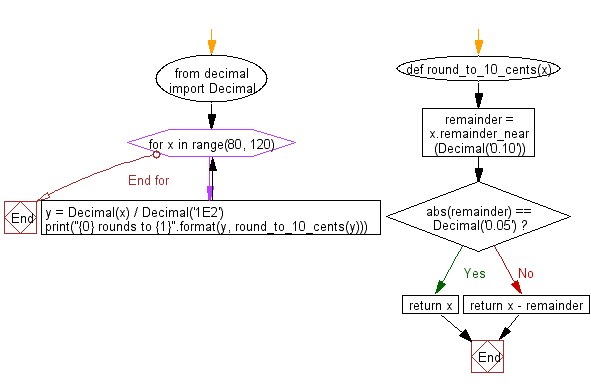﻿ Python: Round a Decimal value to the nearest multiple of 0.10, unless already an exact multiple of 0.05 - w3resource# Python: Round a Decimal value to the nearest multiple of 0.10, unless already an exact multiple of 0.05

## Python module: Exercise-3 with Solution

Write a Python program to round a Decimal value to the nearest multiple of 0.10, unless already an exact multiple of 0.05. Use decimal.Decimal

Sample Solution:

Python Code:

``````from decimal import Decimal
#Source: https://bit.ly/3hEyyY4

def round_to_10_cents(x):
remainder = x.remainder_near(Decimal('0.10'))
if abs(remainder) == Decimal('0.05'):
return x
else:
return x - remainder

# Test code.
for x in range(80, 120):
y = Decimal(x) / Decimal('1E2')
print("{0} rounds to {1}".format(y, round_to_10_cents(y)))
```
```

Sample Output:

```0.80 rounds to 0.80
0.81 rounds to 0.80
0.82 rounds to 0.80
0.83 rounds to 0.80
0.84 rounds to 0.80
0.85 rounds to 0.85
0.86 rounds to 0.90
0.87 rounds to 0.90
0.88 rounds to 0.90
0.89 rounds to 0.90
0.90 rounds to 0.90
0.91 rounds to 0.90
0.92 rounds to 0.90
0.93 rounds to 0.90
0.94 rounds to 0.90
0.95 rounds to 0.95
0.96 rounds to 1.00
0.97 rounds to 1.00
0.98 rounds to 1.00
0.99 rounds to 1.00
1.00 rounds to 1.00
1.01 rounds to 1.00
1.02 rounds to 1.00
1.03 rounds to 1.00
1.04 rounds to 1.00
1.05 rounds to 1.05
1.06 rounds to 1.10
1.07 rounds to 1.10
1.08 rounds to 1.10
1.09 rounds to 1.10
1.10 rounds to 1.10
1.11 rounds to 1.10
1.12 rounds to 1.10
1.13 rounds to 1.10
1.14 rounds to 1.10
1.15 rounds to 1.15
1.16 rounds to 1.20
1.17 rounds to 1.20
1.18 rounds to 1.20
1.19 rounds to 1.20
```

Flowchart:## Visualize Python code execution:

The following tool visualize what the computer is doing step-by-step as it executes the said program:

Python Code Editor:

Have another way to solve this solution? Contribute your code (and comments) through Disqus.

What is the difficulty level of this exercise?

Test your Python skills with w3resource's quiz

﻿

## Python: Tips of the Day

For-else construct useful when searched for something and find it:

```# For example assume that I need to search through a list and process each item until a flag item is found and
# then stop processing. If the flag item is missing then an exception needs to be raised.

for i in mylist:
if i == theflag:
break
process(i)
else:
raise ValueError("List argument missing terminal flag.")
```